# Energy and exergy analysis of flat plate solar collector for three working fluids, under the same conditions

Energy and exergy analysis of flat plate solar collector for three working fluids, under the same conditions

Maghsoud A. Haghghi Seyed M. Pesteei

Faculty of Mechanical Engineering, Urmia University, Urmia, Iran

Corresponding Author Email:
sm.pesteei@urmia.ac.ir
Page:
1-9
|
DOI:
https://doi.org/10.18280/psees.010101
3 August 2017
|
Accepted:
28 August 2017
|
Published:
31 December 2017
| Citation

OPEN ACCESS

Abstract:

The growth and expansion of the population, has caused increased the use of energy in the last few years. One of the cleanest and renewable sources of the energy is the solar energy. The solar energy can be collected by solar collectors. One of the solar collectors is the flat plate solar collector (FPC), that it is used in domestic utilization.

Use of various Nano-fluids to improve the thermal properties of solar collectors, considered as one of the most effective method to optimize the flat plate collectors. In this study, a FPC in terms of energy and exergy, for three fluids (water, air and TiO2 Nano-fluid) have been investigated. According to the results obtained and under the same conditions, destruction exergy of water is more than other two fluids and TiO2 Nano-fluid has the least amount of destruction exergy. Also, by increasing in the total radiation on tilted surface (Gt) TiO2 Nano-fluid’s exergy efficiency is more than the other fluids in this study. By increasing ambient temperature, the exergy efficiency decreases, that water has the most variation.

Due to the temperature range of the inlet working fluid to the collector’s tubes, observed that outlet temperature of the TiO2 Nano-fluid is about 50°C higher than when water enters it. Therefore, the initial statement about Nano-fluids is confirmed. In appropriate conditions, the collector’s efficiency is between 45% - 50%, thus FPC is one of the best devices for domestic utilization.

Keywords:

solar energy, flat plate solar collector, water, air, Tio2 nano-fluid, exergy

1. Introduction

Solar energy is one of the clean and renewable energy that it is gratis. Therefore, using it will save wealth and end the dangers of emissions from fossil fuels.

For various purposes, in this regard different collectors designed and built. One of them is flat plate solar collector that it is stationary and its concentration rate is one. It is commonly used in residential buildings.

In domestic utilization based on solar energy systems, the flat plate solar collector is the system’s main part. FPC is a heat exchanger that, receives solar energy and then gives it to the working fluid that flows inside its tubes. By doing this, it will increase working fluid’s energy at FPC’s outlet. The effort is that the outlet fluid has a lot of energy. Therefore, a lot of scientific work has been done to investigate the FPC. These studies, aimed at understanding the factors affecting the performance of FPC, in order to build high quality collectors. So, energy and exergy analysis for FPC is very important.

In refs. [1-6] energy and exergy analysis methods, for flat plate solar collectors, such as energy and exergy efficiency, destruction exergy, working fluid’s outlet status are elaborated.

Akio Suzuki [2-3] discussed the general theory of exergy balance analysis and application to solar collectors. His work presented the exact relations for the solution of the solar collector's exergy equations. A.K. Kara  did research about exergy optimization of flow rates in flat plate solar collectors.

Geng Liu et al.  suggested a methodology for calculating the delivered and destruction exergy by the operation of a solar heating system. He showed that, radiation and convection heat transfer inside the solar collector and conversion of solar energy to thermal energy, extremely effective on destruction exergy.

I. Luminosu and L. Fara  discussed about the optimal operation mode of a flat solar collector by exergy analysis and numerical simulation.

S.Farahat et al. [11-12] did exergetic optimization of flat plate solar collectors based on water working fluid, and showed the factors affecting the exergy efficiency such as design parameters, ambient and working fluid’s inlet temperature, total solar radiation and etc.

Farzad Jafarkazemi, Emad Ahmadifard  discussed about energy and exergy evaluation of flat plate solar collectors and showed that designing the FPC’s system, is which inlet water temperature is approximately 40 ℃ more than the ambient temperature as well as a lower flow rate will enhance the system’s total performance.

Also, from different Nano-fluids used in the FPC [19-21] and then calculated its performance. many studies [17-21] have been done to determine Nano-fluid’s properties.

In the previous researches, provided exact equation for energy and exergy calculations. Given the importance of using solar collectors, and increasing demand in this case, energy and exergy analysis of these systems are very importance. Therefore, in this paper from three different fluids which are water, air and TiO2 Nano-fluid, under the same conditions, will be used as the working fluid entering the collector. Also, energy and exergy of FPC will be compared in all three cases.

2. Modeling

2.1. Energy analysis

Collector’s energy analysis, in order to attain the amount of heat it receives, obtained its efficiency and working fluids outlet temperature is very important.

For this purpose, the climatic conditions of the place where the collector (such as solar radiation received, solar radiation angle, Sunset angle, latitude angle and etc.) is installed must be available.

By knowing the amount of monthly average solar radiation received on the horizontal surface of the earth, monthly average diffuse radiation calculated from the equation 1 :

$\overline{\mathrm{H}}_{\mathrm{d}}=\overline{\mathrm{H}}_{\mathrm{b}}\left(1-1.13 \overline{\mathrm{K}}_{\mathrm{t}}\right)$    (1)

$\overline{\mathrm{H}}_{\mathrm{d}}$ is monthly average diffuse radiation, $\overline{\mathrm{H}}_{\mathrm{b}}$ is monthly average radiation received on the horizontal surface of the ground, $\overline{\mathrm{K}}_{\mathrm{t}}$ is  monthly average clearness index.

To obtain the amount of monthly average absorbed solar radiation by FPC, (2-14) steps are followed.

At first, according to the incident beam angle and FPC glass, refracted beam angle can be calculated from the equation2 [22-23]:

$\mathrm{n}_{1} \cdot \sin \alpha_{\mathrm{i}}=\mathrm{n}_{2} \cdot \sin \theta$    (2)

n1 and n2 are refracted index for air and glass respectively, αi is incident beam angle and θ is refracted beam angle.

Transmittance absorption losses factor is [22-23]:

τα = exp($\frac{-\mathrm{KL}}{\cos \theta}$)    (3)

KL is absorbed solar radiation rate for glass

Then transmittance refraction losses factor is [22-23]:

τr = $\frac{1}{2}\left(\frac{1-r \|}{1+r \|}+\frac{1-r \perp}{1+r \perp}\right)$        (4)

In this equation $\mathbf{r}_{ \|}$ and $\mathrm{r}_{\perp}$ are parallel and perpendicular component of unpolarized radiation respectively and can be calculated from equations 5 and 6 [22-23]:

$\mathbf{r}_{ \|}=\frac{\tan ^{2}(\theta-\alpha)}{\tan ^{2}(\theta+\alpha)}$        (5)

$r_{\perp}=\frac{\sin ^{2}(\theta-\alpha)}{\sin ^{2}(\theta+\alpha)}$        (6)

Therefore, transmittance factor for glass is [22-23]:

τ = τα × τr    (7)

At this step, absorptance factor can be found from the properties of the absorber, which is [22-23]:

$\frac{\alpha_{\mathrm{B}}}{\alpha_{\mathrm{n}}}=1+2.0345 \times 10^{-3} \alpha-1.99 \times 10^{-4} \alpha^{2}+5.324 \times$$10^{-6} \alpha^{3}-4.799 \times 10^{-8} \alpha^{4} (8) \alpha_{\mathrm{n}} is absorptance factor at normal incident can be found from the properties of the absorber. The amount of effective product transmittance –absorptance that finally absorbs the absorber is : (\tau \alpha)_{\mathrm{B}}=(1.01 \tau) . \alpha_{\mathrm{B}} (9) For given collector tilted angle (β), the effective incidence angle for diffuse radiation from sky and effective incidence angle for ground reflected radiation, can be calculated from equations 10 and 11 respectively [22-23]: \theta_{\mathrm{e}, \mathrm{D}}=59.68-0.1388 \beta+0.001497 \beta^{2} (10) \theta_{\mathrm{e}, \mathrm{G}}=90-0.5788 \beta+0.00269 \beta^{2} (11) By placing \theta_{\mathrm{e}, \mathrm{D}} and \theta_{\mathrm{e}, \mathrm{G}} in the equation 2 and solving equations number 2 to 9, the effective product transmittance – absorptance that finally will be diffused and the effective product transmittance – absorptance that finally reflected from the ground can be calculated from equations 12 and 13 respectively [22-23]: (\tau \alpha)_{\mathrm{D}}=(1.01 \tau) . \alpha_{\mathrm{D}} (12) (\tau \alpha)_{\mathrm{G}}=(1.01 \tau) . \alpha_{\mathrm{G}} (13) Table 1. Flat plate solar collector features  value sign 2 (m2) Ac 0.91 αn 20 (degree) αi 30 (degree) β 0.012 (m) Di 0.014 (m) D 0.9 εP 0.88 εg 0.004 δg 0.04 δe 0.005 δP 320 (w/m2·K) hfi 0.05 (W/m·K) KP 385 (W/m·℃) Ka 0.04 KL 2 (m) Lr 0.01 (Kg/s) \dot{\mathrm{m}} 1 Ng 7 NT 1 n1 1.526 n2 100 (Kpas) Pi 280(K) Ta 340 (K) TP 298.15 Ti 0.12 (m) Wi 10 (m/s) V 1 (m/s) Vel Therefore, monthly average absorbed solar radiation by FPC is [22-23]: \overline{\mathrm{S}}=\overline{\mathrm{H}}_{\mathrm{b}} \cdot \overline{\mathrm{R}}_{\mathrm{b}} \cdot (\tau \alpha)_{\mathrm{B}}+(\mathrm{\tau} \alpha)_{\mathrm{D}} \cdot\left(\frac{1+\cos \beta}{2}\right) \cdot \overline{\mathrm{H}}_{\mathrm{d}}$$+\rho_{\mathrm{G}} .(\tau \alpha)_{\mathrm{G}} \cdot\left(\frac{1-\cos \beta}{2}\right) \cdot\left(\overline{\mathrm{H}}_{\mathrm{b}}+\overline{\mathrm{H}}_{\mathrm{d}}\right)$    (14)

$\overline{\mathrm{R}}_{\mathrm{b}}$ is monthly beam radiation tilt factor, ρG is ground reflectance factor.

Monthly average total solar radiation is [22-23]:

$\overline{\mathrm{I}}_{\mathrm{t}}=\frac{\overline{\mathrm{s}}}{(\mathrm{\tau} \alpha) \mathrm{ave}}$    (15)

$(\tau \alpha)_{\text { ave }}$ is average effective product transmittance – absorptance and can be calculated from equation16 [22-23]:

$(\tau \alpha)_{\mathrm{ave}}=0.96(\tau \alpha)_{\mathrm{B}}$    (16)

By calculated overall heat loss coefficient, the rate of useful energy collected from FPC and FPC’s efficiency obtained. overall heat loss coefficient, include three terms:

1. top loss coefficient :

$\mathrm{U}_{\mathrm{t}}=\frac{1}{\frac{\mathrm{Ng}}{\frac{\mathrm{C}}{\mathrm{T} \mathrm{p}}\left(\frac{\mathrm{T}_{\mathrm{p}}-\mathrm{T}_{\mathrm{a}}}{\mathrm{N} \mathrm{g}+\mathrm{f}}\right)^{0.33}+\frac{1}{\mathrm{h}_{\mathrm{W}}}}}$            （17）

Ng is number of glass cover, Tp is mean absorber temperature, Ta is ambient air temperature, hw is wind convection heat loss coefficient, C and f are constant parameters .

$\mathrm{h}_{\mathrm{w}}=\frac{8.6 \mathrm{V}^{0.6}}{\mathrm{L}^{0.4}}$    (18)

$\mathrm{C}=365.9\left(1-0.00883 \beta+0.0001298 \beta^{2}\right)$    (19)

$\mathrm{f}=\left(1-0.04 \mathrm{h}_{\mathrm{w}}+0.0005 \mathrm{h}_{\mathrm{w}}^{2}\right)\left(1+0.091 \mathrm{N}_{\mathrm{g}}\right)$    (20)

2. bottom heat loss coefficient :

$\mathrm{U}_{\mathrm{b}}=\frac{1}{\frac{\mathrm{tb}}{\mathrm{k}_{\mathrm{b}}}+\frac{1}{\mathrm{h}_{\mathrm{c}, \mathrm{b}-\mathrm{a}}}}$            (21)

tb is thickness of back insulation, hc,b-a is convection heat loss coefficient from back to ambient, Kb is conductivity of back insulation.

3. heat loss coefficient from the collector edge :

$\mathrm{U}_{\mathrm{e}}=\frac{1}{\frac{\mathrm{t}_{\mathrm{e}}}{\mathrm{k}_{\mathrm{e}}}+\frac{1}{\mathrm{h}_{\mathrm{c}, \mathrm{e}-\mathrm{a}}}}$            (22)

te is thickness of edge insulation, hc,e-a  is convection heat loss coefficient from edge to ambient, Ke is conductivity of edge insulation.

Therefore, overall heat loss coefficient is [11-12]:

UL = Ut + Ue + Ub                                                               (23)

The useful heat gain by the working fluid is :

$\mathrm{Q}_{\mathrm{u}}=\dot{\mathrm{m}} \cdot \mathrm{C}_{\mathrm{p}} \cdot\left(\mathrm{T}_{\mathrm{out}}-\mathrm{T}_{\mathrm{in}}\right)$    (24)

$\dot{\mathrm{m}}$ is working fluid mass rate, Cp is heat capacity, Tout and Tin are outlet and inlet temperature respectively.

The useful heat gain of FPC system, considering the heat losses from the FPC to the atmosphere, is [11,22]:

$\mathrm{Q}_{\mathrm{u}}=\mathrm{A}_{\mathrm{c}}\left[\mathrm{G}_{\mathrm{t}}(\tau \alpha)-\mathrm{U}_{\mathrm{L}}\left(\mathrm{T}_{\mathrm{p}}-\mathrm{T}_{\mathrm{a}}\right)\right]$    (25)

Ac is collector area, Gt is total solar radiation.

Collector's performance, collector efficiency and collector efficiency factor calculated from equations 27 and 28 :

$\eta=\frac{\mathrm{Qu}}{\mathrm{A}_{\mathrm{C}} \cdot \mathrm{G}_{\mathrm{t}}} \times 100$    (26)

$\mathrm{F}_{\mathrm{C}}=\frac{\frac{1}{\mathrm{U}_{1}}}{\mathrm{W}_{\mathrm{i}}\left[\frac{1}{\mathrm{U}_{1} \cdot\left(\mathrm{D}+\left(\mathrm{W}_{\mathrm{i}}-\mathrm{D}\right) \cdot \mathrm{F}_{\mathrm{f}}\right)}+\frac{1}{\mathrm{C}_{\mathrm{b}}}+\frac{1}{\mathrm{\pi} \cdot \mathrm{D}_{\mathrm{i}} \cdot \mathrm{h}_{\mathrm{fi}}}\right]}$               (27)

Wi is tubes spacing, D is tube outside diameter, Di is tube inside diameter, hfi is heat transfer coefficient inside of the tube, Ff is fin efficiency, Cb is bond conductance.

Fin efficiency is [22-23]:

$\mathrm{F}_{\mathrm{f}}=\frac{\tanh \left(\mathrm{X}\left(\frac{\mathrm{w}_{\mathrm{i}-\mathrm{D}}}{2}\right)\right)}{\mathrm{x}\left(\frac{\mathrm{W}_{\mathrm{i}}-\mathrm{D}}{2}\right)}$            (28)

X is a constant parameter and equal to [22-23]:

$X=\left[\frac{U_{1}}{K \delta_{t}}\right]$    (29)

Plate’s material is copper. K is plate heat transfer coefficient, $\delta_{\mathrm{t}}$ is plate thickness.

Bond conductance can be calculated from equation 31 [22-23]:

$\mathrm{C}_{\mathrm{b}}=\frac{\mathrm{K}_{\mathrm{b}} \cdot \mathrm{b}}{\gamma}$    (30)

Kb is bond thermal conductivity, b is bond width, γ is bond thickness.

The fluid outlet temperature has a very important role in collector systems. In FPC it is equal to :

$\mathrm{T}_{\mathrm{out}}=\mathrm{T}_{\mathrm{in}}+\left[\frac{1}{\mathrm{U}_{1}}\left(\overline{\mathrm{S}}_{\mathrm{w}}-\mathrm{U}_{1}\left(\mathrm{T}_{\mathrm{in}}-\mathrm{T}_{\mathrm{a}}\right)\right)\right]$$\cdot\left[1-\exp \left(\frac{-\mathrm{A}_{\mathrm{c}} \cdot \mathrm{U}_{\mathrm{L}} \cdot \mathrm{F}_{\mathrm{c}}}{\dot{\mathrm{m}} \cdot \mathrm{C}_{\mathrm{p}}}\right)\right] (31) \overline{\mathrm{S}}_{\mathrm{w}} is average absorbed solar radiation in (W/m2)  \overline{\mathrm{S}}_{\mathrm{w}}=\frac{\overline{\mathrm{G}}_{\mathrm{t}}}{(\tau \alpha) \mathrm{ave}} (32) 2.2 Exergy analysis Exergy analysis is a method that use the second law of thermodynamics for the analysis, design and improvement of energy. Exergy is defined as the maximum amount of power which can be produced by a system and has an important role in thermodynamic analysis. Exergy analysis for a control volume can be calculated from equation 34 : \frac{\mathrm{dEX}_{\mathrm{cv}}}{\mathrm{dt}}=\sum_{\mathrm{j}}\left(1-\frac{\mathrm{T}_{0}}{\mathrm{T}_{\mathrm{j}}}\right) \dot{\mathrm{Q}}_{\mathrm{j}}-\left(\dot{\mathrm{W}}_{\mathrm{cv}}-\mathrm{P}_{0} \frac{\mathrm{d} \mathrm{V}_{\mathrm{cv}}}{\mathrm{dt}}\right)+$$\sum \dot{\mathrm{m}}_{\mathrm{i}} \mathrm{EX}_{\mathrm{i}}-\sum \dot{\mathrm{m}}_{\mathrm{e}} \mathrm{EX}_{\mathrm{e}}-\dot{\mathrm{EX}}_{\mathrm{d}}$    (33)

When FPC system is in a steady state, exergy balance is [2,14]:

$\dot{\mathrm{EX}}_{\mathrm{in}}-\dot{\mathrm{EX}}_{\mathrm{out}}-\dot{\mathrm{EX}}_{\mathrm{L}}-\dot{\mathrm{EX}}_{\mathrm{d}}=0$    (34)

1.inlet exergy:

Exergy flows into a system includes two terms, inlet exergy with mass flow and inlet exergy with solar radiation absorbed by the collector.

a. Inlet exergy with mass flow is [2,11]:

$\dot{\mathrm{EX}}_{\mathrm{in}, \mathrm{f}}=\dot{\mathrm{m}} \cdot \mathrm{C}_{\mathrm{p}}\left(\mathrm{T}_{\mathrm{in}}-\mathrm{T}_{\mathrm{a}}-\left(\mathrm{Ta} \ln \frac{\mathrm{T}_{\mathrm{in}}}{\mathrm{T}_{\mathrm{a}}}\right)\right)+\frac{\dot{\mathrm{m}} \Delta \mathrm{P}_{\mathrm{in}}}{\rho}$    (35)

$\Delta \mathrm{P}_{\mathrm{in}}$ is the pressure difference of the working fluid with the surroundings at FPC’s inlet.

b. Inlet exergy with solar radiation absorbed by the collector is :

$\dot{\mathrm{EX}}_{\mathrm{in}, \mathrm{q}}=\eta_{\mathrm{o}} . \mathrm{G}_{\mathrm{t}} \cdot \mathrm{A}_{\mathrm{c}}\left(1-\frac{\mathrm{T}_{\mathrm{a}}}{\mathrm{T}_{\mathrm{S}}}\right)$    (36)

Ts is Apparent solar temperature, ηo is optical efficiency and equal to [15,16]:

ηo $=\frac{\overline{\mathrm{S}}}{\overline{\mathrm{I}}_{\mathrm{t}}}$    (37)

Thus, inlet exergy is [2-3]:

$\dot{\mathrm{EX}}_{\mathrm{in}}=\dot{\mathrm{EX}}_{\mathrm{in}, \mathrm{f}}+\dot{\mathrm{EX}}_{\mathrm{in}, \mathrm{q}}$    (38)

2. outlet exergy:

The outlet exergy includes only the outlet exergy with mass flow and equal to [2,7]:

$\dot{\mathrm{EX}}_{\mathrm{out}}=\dot{\mathrm{m}} \cdot \mathrm{C}_{\mathrm{p}}\left(\mathrm{T}_{\mathrm{out}}-\mathrm{T}_{\mathrm{a}}-\left(\mathrm{Ta} \ln \frac{\mathrm{T}_{\mathrm{out}}}{\mathrm{T}_{\mathrm{a}}}\right)\right)+\frac{\dot{\mathrm{m}} \cdot \Delta \mathrm{P}_{\mathrm{out}}}{\rho}$    (39)

$\Delta \mathrm{P}_{\mathrm{out}}$ is the pressure difference of the working fluid with the surroundings at FPC’s outlet.

3. Leakage exergy

Includes heat leakage from the absorber plate to the environment. The outlet exergy is the desired exergy and the exergy leakage equals the undesired exergy losses.

$\dot{\mathrm{EX}}_{\mathrm{L}}=\mathrm{U}_{\mathrm{L}} \cdot \mathrm{A}_{\mathrm{c}}\left(\mathrm{T}_{\mathrm{p}}-\mathrm{T}_{\mathrm{a}}\right)\left(1-\frac{\mathrm{T}_{\mathrm{a}}}{\mathrm{T}_{\mathrm{p}}}\right)$    (40)

4. Destruction exergy:

Destruction exergy includes three terms which is discussed below.

a. Destruction exergy due to pressure drop of inside the tube [2,26]:

$\dot{\mathrm{EX}}_{\mathrm{d}, \Delta \mathrm{P}}=\left(\frac{\dot{\mathrm{m}} \Delta \mathrm{P}}{\rho}\right)\left(\frac{\mathrm{T}_{\mathrm{a}} \ln \frac{\mathrm{T}_{\mathrm{out}}}{\mathrm{Ta}}}{\mathrm{T}_{\mathrm{out}}-\mathrm{T} \mathrm{in}}\right)$    (41)

$\Delta \mathrm{P}=\rho \cdot \mathrm{g} \cdot \left(\mathrm{L}_{\mathrm{r}} \cdot \sin \beta+\mathrm{h}_{1}\right)$    (42)

g is gravity acceleration, Lr is tube length, h1 is total pressure drop and equal to :

$\mathrm{h}_{1}=\frac{8 \cdot \dot{\mathrm{m}}^{2}}{\mathrm{n}_{\mathrm{r}} \cdot \mathrm{g} \cdot \rho^{2} \cdot \pi^{2} \cdot \mathrm{D}_{\mathrm{i}}^{4}}\left(\mathrm{f} \frac{\mathrm{L} \mathrm{r}}{\mathrm{D}_{\mathrm{i}}}+\sum_{\mathrm{i}=1}^{\mathrm{n}_{\mathrm{r}}} \mathrm{K}_{\mathrm{L}}\right)$    (43)

nr is number of tube, KL is partial pressure drop coefficient of connections that in tube’s inlet equal to 1 and at outlet equal to 0.5, f is friction coefficient and obtained from equation 45 :

$\left\{\begin{array}{ll}{\mathrm{f}=\frac{64}{\mathrm{Re}}} & {\text { laminar flow }} \\ {\mathrm{f}=\frac{0.079}{\mathrm{Re}^{0.25}}} & {\text { turbulent flow }}\end{array}\right.$    (44)

b. Destruction exergy due to solar temperature difference with absorber plate surface [2-3]:

$\dot{\mathrm{EX}}_{\mathrm{d}, \Delta \mathrm{T}_{\mathrm{S}}}=\eta_{\mathrm{o}} \cdot \mathrm{G}_{\mathrm{t}} \cdot {\mathrm{A}_{\mathrm{p}}} \cdot \mathrm{T}_{\mathrm{a}} \cdot\left(\frac{1}{\mathrm{T}_{\mathrm{p}}}-\frac{1}{\mathrm{T}_{\mathrm{S}}}\right)$    (45)

c. Destruction exergy due to the temperature difference between absorber plate surface and working fluid that is [2,7]:

$\dot{\mathrm{EX}}_{\mathrm{d}, \Delta \mathrm{P}_{\mathrm{f}}}=\dot{\mathrm{m}} \cdot \mathrm{C}_{\mathrm{p}} \cdot \mathrm{T}_{\mathrm{a} \cdot}\left(\ln \frac{\mathrm{T}_{\mathrm{out}}}{\mathrm{T}_{\mathrm{in}}}-\frac{\mathrm{T}_{\mathrm{out}}-\mathrm{T}_{\mathrm{in}}}{\mathrm{T}_{\mathrm{p}}}\right)$

=$1-\left\{\left(1-\eta_{O}\right)+\frac{\dot{m} \Delta P}{\rho G_{t}\left(1-\frac{T_{a}}{T_{s}}\right)} \frac{T_{a} \ln \left(\frac{T_{o u t}}{T_{a}}\right)}{\left(T_{o u t}-T_{i n}\right)}+\right.$    (46)

Therefore, destruction exergy is [2,3,7]:

$\dot{\mathrm{EX}}_{\mathrm{d}}=\dot{\mathrm{EX}}_{\mathrm{d}, \Delta \mathrm{P}}+\dot{\mathrm{EX}}_{\mathrm{d}, \Delta \mathrm{T}_{\mathrm{S}}}+\dot{\mathrm{EX}}_{\mathrm{d}, \Delta \mathrm{P}_{\mathrm{f}}}$    (47)

By doing the balance of exergy, exergy efficiency to understand the FPC performance is defined, that is [11,15]:

$\eta_{\mathrm{EX}}$=$\frac{\dot{\mathrm{m}}\left[\mathrm{C}_{\mathrm{p}}\left(\mathrm{T}_{\mathrm{out}}-\mathrm{T}_{\mathrm{in}}-\left(\mathrm{T}_{\mathrm{a}} \cdot \ln \frac{\mathrm{T}_{\mathrm{out}}}{\mathrm{T}_{\mathrm{i}}}\right)\right)-\frac{\Delta \mathrm{P}}{\rho}\right]}{\mathrm{G}_{\mathrm{t}} \cdot \mathrm{A}_{\mathrm{p}} \cdot\left(1-\frac{\mathrm{T} \mathrm{a}}{\mathrm{T}_{\mathrm{S}}}\right)}$$=$$1-\left\{\left(1-\eta_{O}\right)+\frac{\dot{m} \Delta P}{\rho G_{t}\left(1-\frac{T_{a}}{T_{s}}\right)} \frac{T_{a} \ln \left(\frac{T_{o u t}}{T_{a}}\right)}{\left(T_{o u t}-T_{i n}\right)}+\right.$$\frac{\eta_{O} T_{a}}{\left(1-\frac{T_{a}}{T_{S}}\right)}\left(\frac{1}{T_{P}}-\frac{T_{a}}{T_{s}}\right)+\frac{U_{l}\left(T_{P}-T_{a}\right)}{G_{t}\left(1-\frac{T_{a}}{T_{S}}\right)}\left(1-\frac{T_{a}}{T_{P}}\right)+$$\frac{\dot{m} C_{P} T_{a}}{G_{t} A_{c}} \frac{\left(\ln \left(\frac{T_{o u t}}{T_{i n}}\right)-\frac{\left(T_{o u t}-T_{i n}\right)}{T_{P}}\right)}{\left(1-\frac{T_{a}}{T_{S}}\right)} \}$    (48)

3. Thermo-physical Characteristics of the Nano-fluid

Nano-fluids are liquid suspensions of particles that one of their particles dimensions smaller than 100 nm. Nano-fluid that in this study is TiO2 Nano-fluid, considered as powder that an average particle’s diameter is 15 nm, which adds to the base fluid (water) to improve its thermal properties.

Density of Nano-fluid is :

ρnf = φv·ρnp + (1-φv)·ρbf    (49)

φv is volume concentrations, ρnp and ρbf are density of nano powder and base fluid (water) respectively.

Thermal capacity of Nano-fluid is :

(ρ·Cp)nf = φv·(ρ·Cp)np + (1-φv)·(ρ·Cp)bf    (50)

Cp,np and Cp,bf are thermal capacity of nano powder and base fluid respectively.

And viscosity of Nano-fluid is :

μnf  = μbf (1+2.5 φv +6.5 φv2)    (51)

Table 2.  TiO2 Nano powder properties

 φv(%) Cp (j/kg·K) ρ (kg/m3) Nano powder 0.99 4182 997.1 TiO2

Table 3. Working fluids properties

 μ(Pa·s) Cp (j/kg·K) ρ (kg/m3) fluid 890.5 × 10 -6 4182 997.1 Water 18.45 × 10 -6 1007 1.72 Air

4. Result and Discussion

In this study, for a flat plate solar collector that its features are defined, by using three fluids that are water, air and TiO2 Nano-fluid under the same condition energy and exergy equations are modeling and solving.

As it seen in table 4 the values obtained from energy analysis are reported:

Table 4. Energy analysis result for FPC

 value parameter 20 Mj/M2 $\overline{\mathrm{H}}_{\mathrm{b}}$ 6.44 Mj/M2 $\overline{\mathrm{H}}_{\mathrm{d}}$ 0.6 $\overline{\mathrm{K}}_{\mathrm{t}}$ 0.8052 ($\tau \alpha$)B 0.7099 ($\tau \alpha$)D 0.402 ($\tau \alpha$)G 0.773 ($\tau \alpha$)ave 0.9064 $\alpha_{\mathrm{B}}$ 0.8639 $\alpha_{\mathrm{D}}$ 0.6914 $\alpha_{\mathrm{G}}$ 12.95 Degree θ 56.86 Degree θe,D 75.06 Degree θe,G 36.84 Mj/M2 $\overline{\mathrm{S}}$ 47.65 Mj/M $\overline{\mathrm{I}}_{\mathrm{t}}$ 5.276 W/m2 . K Ul 3.076 W/m2 .K Ut 0.5 W/m2 .K Ub 1.6 W/m2 .K Ue 29.95 W/m2 .K hw 1018 W Qu 1000 W/m2 Gt 0.9254 FC 0.9691 Ff 5.853 X 1293.66 W/m2 $\overline{\mathrm{S}}_{\mathrm{w}}$

## 1.pngFigure 1. Effects of ambient temperature to FPC efficiency at variable total solar radiation.

As it seen in fig. 1, effect of ambient temperature is shown in the total solar radiation range between 500-1200 Watts. It can be interpreted that efficiency of FPC is directly related to ambient temperature So that, by increasing ambient temperature and total solar radiation FPC Efficiencies increases. Approximately FPC Efficiencies at an appropriate collector tilt angle and working fluid mass flow rate during the year is between 45 % – 55% that is a suitable range.

As it seen in fig. 2, for working fluids that its inlet temperature is between 290 – 320 kelvins, outlet temperature is obtained. TiO2 Nano-fluid has the highest outlet temperature that its outlet temperature approximately (70-80) ℃ higher than water and (30-40) ℃ higher than air. therefore, Nano-fluids has the more ability than other working fluids.

## 2.png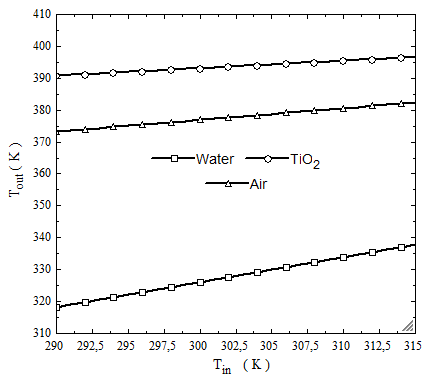Figure 2. Working fluids outlet temperature, based on their inlet temperature

## 3.png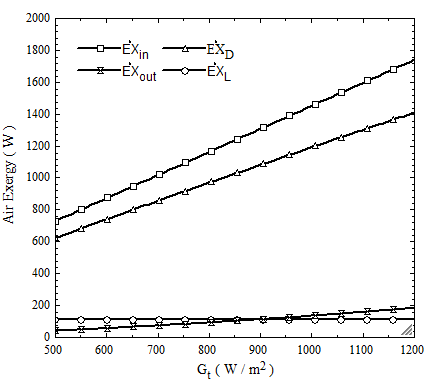Figure 3. Exergy of water, based on total solar radiation

In fig.3, fig.4 and fig.5 effect of total radiation on working fluids exergy, in range between 500-1200 (W/m2) is discussed.

According to the obtained diagrams, when total solar radiation is increasing, exergy increases. Destruction exergy is very affected from solar radiation when ambient temperature is constant. Inlet exergy of working fluids is very higher than outlet exergy.

## 4.pngFigure 4. Exergy of TiO2 Nano-fluid, based on total solar radiation

Generally, inlet exergy is between 700-1700 watts, outlet exergy is between 80-200 watts, leakage exergy is between 100-150 watts and destruction exergy is between 600-1400 watts. Exergy of TiO2 Nano-fluid in the same condition is higher than air, and water has the least exergy.

## 5.png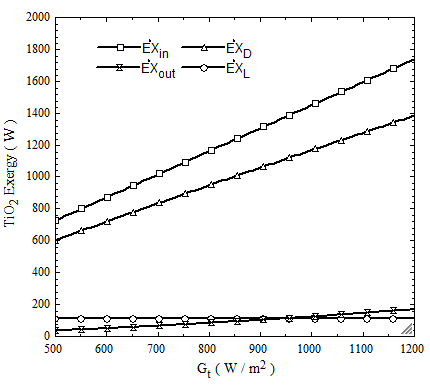Figure 5. Exergy of air Nano-fluid, based on total solar radiation

## 6.png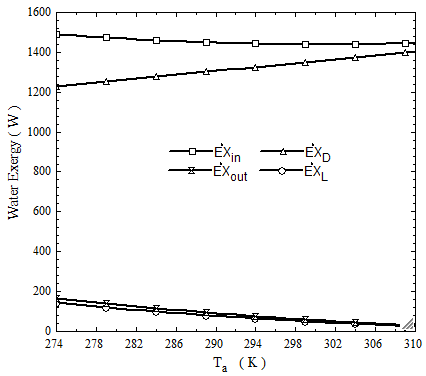Figure 6. Exergy of water, based on ambient temperature

As it seen in fig. 6, fig. 7 and fig. 8 effect of ambient temperature on working fluids exergy, in range between 274-310 kelvins is discussed.

As the ambient temperature increases, the inlet exergy decreases, which water has the most changes and TiO2 Nano-fluids gives the slightest changes in these conditions.

Generally, the range of exergy changes for inlet exergy is between 1400-1500 watts, for outlet exergy is between 180-20 watts, for leakage exergy is between 180-20 watts. But destruction exergy increases and its changes is between 1200-1450 watts.

## 7.pngFigure 7. Exergy of TiO2 Nano-fluid, based on ambient temperature

## 8.png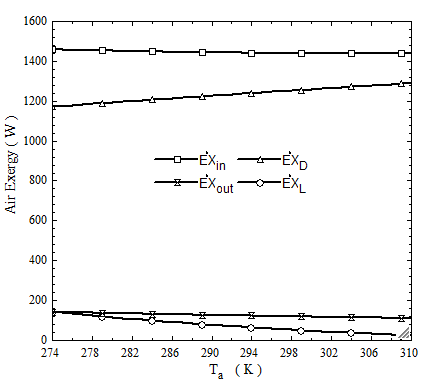Figure 8. Exergy of air, based on ambient temperature

As it seen in fig. 9, destruction exergy of water, more than other two fluids.

Also, the destroyed exergy of the TiO2 Nano-fluid has the lowest value. Therefore, the use of Nano-fluids is more efficient.

In fig. 10, exergy efficiency based on total solar radiation that is between 700-1200 (W/m2) has been shown. By increasing total solar radiation, the exergy efficiency is rising for all three working fluids. However, the increase in total solar radiation has a great impact on exergy efficiency of TiO2 Nano-fluid.

In fig. 12, the effect of the ambient temperature on the exergy efficiency of the working fluids is shown. By increasing ambient temperature, the exergy efficiency is decreased, that water has the most negative variations in exergy efficiency under the same condition. In this state, TiO2 Nano-fluid also has the highest exergy efficiency.

## 9.png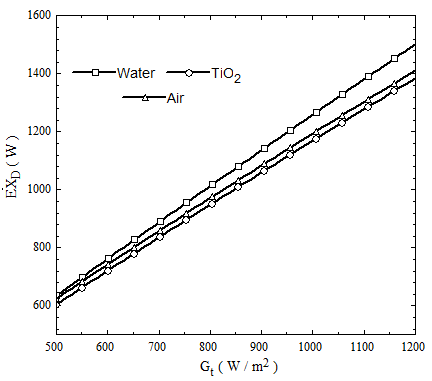Figure 9. Compare destruction exergy of working fluids, based on total solar radiation

## 10.pngFigure 10. Compare exergy efficiency of working fluids, based on total solar radiation

## 11.png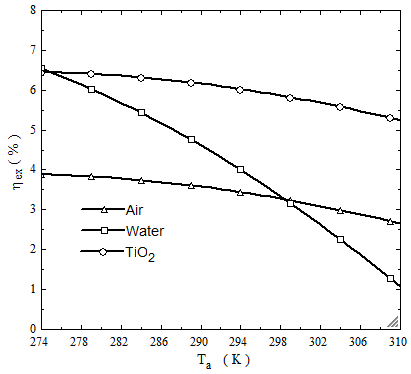Figure 11. Compare exergy efficiency of working fluids, based on ambient temperature

In fig. 12, The effect of increasing the overall heat loss coefficient on the exergy efficiency is shown. As it increases, gradually decreases the exergy efficiency.

In this state, the TiO2 Nano-fluid is more susceptible to overall heat loss than the other two fluids.

In fig. 13, the effect of exergy efficiency on the optical efficiency is shown. It is observed that the TiO2 Nano-fluid is more sensitive than other two fluids.

## 12.pngFigure 12. Compare exergy efficiency of working fluids, based on overall heat loss coefficient

## 13.png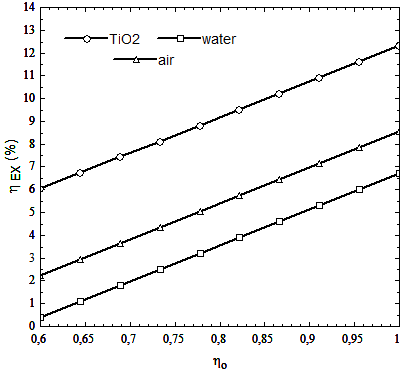Figure 13. Compare exergy efficiency of working fluids, based on overall optical efficiency

5. Conclusions

1. BY increasing total solar radiation, the energy and exergy efficiency are increase. But when the ambient temperature rises, the exergy efficiency decreases.

2. The use of a Nano-fluid working fluid in FPC systems, will increase the temperature at the outlet of collector.

3. The working fluid in the collector entrance, has a large exergy. But in outlet of FPC, it encounters a huge drop in exergy.

4. Destruction exergy in the collector is very high, which results in a sharp drop in the collector's exergy efficiency.

5. The overall loss coefficient in FPC is not a constant parameter and it has a great impact on the exergy efficiency. Therefore, the exact calculation is important to get the exact answer.

7. Optical efficiency is a parameter that has a great impact on the exergy efficiency. By increasing optical efficiency, the magnitude of exergy efficiency increases.

8. At all stages, the use of Nano-fluid showed that it improves the performance of the FPC.

Nomenclature
 A collectors area (m2) Cb bond conductance Cp heat capacity of the fluid (kJ/kg K) D diameter (m) $\dot{\mathrm{EX}}$ exergy (W) f friction coefficient F efficiency factor FPC flat plate collector G total solar radiation (w/m2) h1 total pressure drop h convection heat Transfer coefficient(w/K.m2) $\overline{\mathrm{H}}$ monthly average radiation $\overline{\mathrm{I}}_{\mathrm{t}}$ monthly average total solar radiation (Mj/m2) K conductivity (W/m K) $\overline{\mathrm{K}}_{\mathrm{t}}$ monthly average clearness index KL absorbed solar radiation rate for glass Lr tube length (m) $\dot{\mathrm{m}}$ mass flow rate (kg/s) n refraction index N number P pressure (pas) Q heat transfer (W) $\overline{\mathrm{S}}$ radiation absorbed by collector (Mj/m2) $\overline{\mathrm{S}}_{\mathrm{w}}$ radiation absorbed by collector (W/m2) T temperature (K) U collector loss coefficient (W/m2 K) V speed, velocity (m/s) Vel fluid inlet velocity (m/s) Wi tubes spacing (m) X constant parameter

Greek symbols

 αi incident beam angle (degree) α absorptance factor β collector tilted angle (degree) $\Delta$ difference δ thickness (m) ε emissivity φ volume concentrations γ bond thickness (m) η efficiency $\mu$ viscosity $\tau$ transmittance factor $(\tau \alpha)$ effective product transmittance – absorptance θ refracted beam angle (degree) ρ density

Subscripts

 a ambient ave average α absorption B horizontal surface b bottom, bond, horizontal surface bf base fluid c collector D diffuse d destruction, Diffuse e around the collector, edge, exit EX exergy f fin fi inside of the tube G ground g glass i inlet, inside in inlet L length, overall, leakage n normal nf Nano-fluid np Nano-powder o optical out outlet p plate r refraction s solar T tube t top, total u useful v volume w wind ‖ parallel ⊥ vertical

References

 Bejan A, Kearney DW, Kreith F. (1981). Second law analysis and synthesis of solar collector systems. Journal of Solar Energy Engineering 103/23.

 Suzuki A. (1988). General theory of exergy balance analysis and application to solar collectors. Energy 13: 153-160.

 Suzuki A. (1988). A fundamental equation for exergy balance on solar collectors. Journal of Solar Energy Engineering 110: 102-106.

 Torres-Reyes E, Cervantes-de Gortari JG, Ibarra-Salazar BA, Picon-Nuñez M. (2001). A design method of flat-plate solar collectors based on minimum entropy generation. Exergy Int. J. 1: 46 -52.

 Gunerhan H, Hepbasli A. (2007). Exergetic modeling and performance evaluation of solar water heating systems for building applications. Energy and Buildings 39: 509-516.

 Gupta MK, Kaushik SC. (2008). Exergetic performance evaluation and parametric studies of solar air heater. Energy 33: 1669-1702.

 Kara AK. (1989). Exergy optimization of flow rates in flat plate solar collectors. International journal of Energy Research 13: 317-326.

 Liu G, Cengel YA, Turner RH. (1995). Exergy analysis of a solar heating system. Journal of Solar Energy Engineering 117/249.

 Lauminosu I, Fara L. (2005). Determination of the optimal operation mode of a flat solar collector by exergetic analysis and numerical simulation. Energy 30: 731-747.

 Bakirci K. (2012). General models for optimum tilt angles of solar panels: Turkey case study. Renewable and Sustainable Energy Reviews 16: 6149-6159.

 Farahat S, Sarhaddi F, Ajam H. (2009). Exergetic optimization of flat plate solar collectors. Renewable Energy 34: 1169-1174.

 Ajam H, Farahat S, Sarhaddi F. (2005). Exergetic optimization of solar air heaters and comparison with energy analysis. Int. J. of Thermodynamics 8: 183-190

 Tyagi H, Phelan P, Prasher R. (2009). Predicted efficiency of a low-temperature nanofluid – based direct absorption solar collector. Journal of Solar Energy Engineering 131.

 Akpinar EK, Koçyig F. (2010). Energy and exergy analysis of a new flat-plate solar air heater having different obstacles on absorber plates. Applied Energy 87: 3438-3450.

 Jafarkazemi F, Ahmadifard E. (2013). Energetic and exergetic evaluation of flat plate solar collectors. Renewable Energy 56: 55-63.

 Bakirci K. (2012). General models for optimum tilt angles of solar panels: Turkey case study. Renewable and Sustainable Energy Reviews 6: 6149-6159.

 Krishnamurthy S, Bhattacharya P, Phelan PE. (2006). Enhanced mass transport in Nano-fluids. Nano Letters 6: 419-423.

 Phelan PE, Prasher RS, Rosengarten G, Taylor RA. (2010). Nanoﬂuid-Based Direct Absorption Solar Collector. Journal of Renewable and Sustainable Energy 033102-1.

 Pak BC, Cho Young I. (1998). Hydrodynamic and heat transfer study of dispersed fluid with submicron metallic oxide particles fluids with submicron metallic oxide particles. Experimental Heat Transfer: A Journal of Thermal Energy Generation, Transport, Storage, and Conversion 11(2): 151-170.

 Xuan YM, Roetzel W. (2000). Conceptions for heat transfer correlation of nanofluids. International Journal of Heat and Mass Transfer 43: 3701-3707.

 Batchelor GK. (1977). The effect of Brownian motion on the bulk stress in a suspension of spherical particles. J. Fluid Mech. 83.

 Calogirou A. (2009). Solar energy engineering processes and systems. Elsevier Inc. first edition.

 Duffie JA, Beckman WA. (1982). Solar Engineering of Thermal Processes. New York: Wiley.

 Cengel YA, Boles MA. (2005). Thermodynamics an Engineering Approach. 5th ed. McGraw-Hill.

 White FM. (2003). Fluid Mechanics. 5th, Boston: McGraw-Hill Book Company.

 Cengel YA, Cimbala JM. (2009). Fluid Mechanics: Fundamentals and Applications. second ed. McGraw-Hill Higher Education.